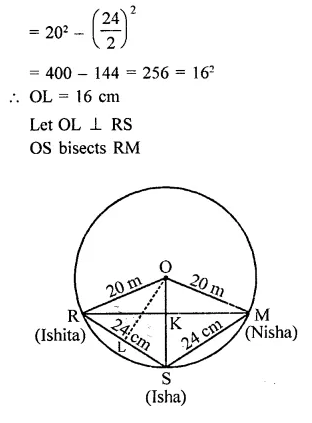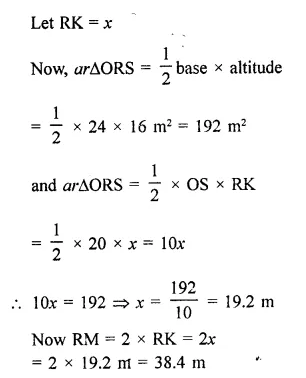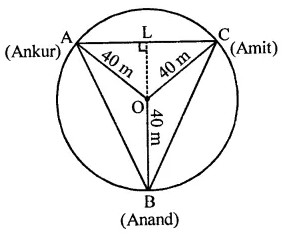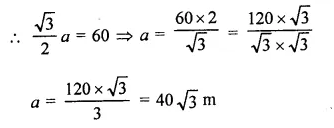# RD Sharma Class 9 Solutions Chapter 15 Areas of Parallelograms and Triangles Ex 15.3

## RD Sharma Class 9 Solutions Chapter 15 Areas of Parallelograms and Triangles Ex 15.3

These Solutions are part of RD Sharma Class 9 Solutions. Here we have given RD Sharma Class 9 Solutions Chapter 15 Areas of Parallelograms and Triangles Ex 15.3

Other Exercises

Question 1.
Three girls Ishita, Isha and Nisha are playing a game by standing on a circle of radius 20 m drawn in a park. Ishita throws a ball to Isha, Isha to Nisha and Nisha to Ishita. If the distance between Ishita and Isha and between Isha and Nisha is 24 m each, what is the distance between Ishita and Nisha. [NCERT]
Solution:
∵ Distance between Isha and Ishita and Ishita and Nisha is same
∴ RS = SM = 24 m
∴They are equidistant from the centre
In right ∆ORL,
OL² = OR² – RL²Hence distance between Ishita and Nisha = 38.4 m

Question 2.
A circular park of radius 40 m is situated in a colony. Three beys Ankur, Amit and Anand are sitting at equal distance on its boundary each having a toy telephone in his hands to talk to each other. Find thelength of the string of each phone. [NCERT]
Solution:
Radius of circular park = 40 m
Ankur, Amit and Anand are sitting at equal distance to each other By joining them, an equilateral triangle ABC is formed produce BO to L which is perpendicular bisector of AC∴ BL = 40 + 20 = 60 m (∵ O is centroid of ∆ABC also)
Let a be the side of ∆ABCHence the distance between each other = 40$$\sqrt { 3 }$$ m

Hope given RD Sharma Class 9 Solutions Chapter 15 Areas of Parallelograms and Triangles Ex 15.3 are helpful to complete your math homework.

If you have any doubts, please comment below. Learn Insta try to provide online math tutoring for you.